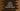# Java program to check if a number is perfect square or not## Java program to check if a number is perfect square or not:

This post will show you how to check if a number is a perfect square or not. It will take one number as input from the user, check if it is a perfect square and print the result.

There are different ways to check for perfect square. Let’s learn these all:

### Example 1: By using Math.sqrt:

If we can express an integer as the square of another integer, this is called a perfect square. For example, 64 is a perfect square as the square of 8 is equal to 64.

The Math.sqrt method is used to find the square root of a number. This method returns a double value. We can convert this value to integer, find the square of that integer value and compare it with the original number to check if the number is a perfect square or not.

``````import java.util.Scanner;

public class Main {
private static boolean isPerfectSquare(int number) {
if (number < 0) return false;

int sqRt = (int) Math.sqrt(number);
return sqRt * sqRt == number;
}

public static void main(String[] args) {
Scanner scanner = new Scanner(System.in);
System.out.println("Enter a number: ");

int no = scanner.nextInt();

if (isPerfectSquare(no)) {
System.out.println(no + " is a perfect square.");
} else {
System.out.println(no + " is not a perfect square.");
}
}
}``````

It will give output as below:

``````Enter a number:
64
64 is a perfect square.

Enter a number:
67
67 is not a perfect square.``````

### Example 2: By using Math.floor and Math.ceil:

We can use Math.floor and Math.ceil on the return value of Math.sqrt(). If the values of both of these methods are equal, we can say that the number is a perfect square. Else, it is not a perfect square.

``````import java.util.Scanner;

public class Main {
private static boolean isPerfectSquare(int number) {
if (number < 0) return false;

double sqRt = Math.sqrt(number);
return Math.floor(sqRt) == Math.ceil(sqRt);
}

public static void main(String[] args) {
Scanner scanner = new Scanner(System.in);
System.out.println("Enter a number: ");

int no = scanner.nextInt();

if (isPerfectSquare(no)) {
System.out.println(no + " is a perfect square.");
} else {
System.out.println(no + " is not a perfect square.");
}
}
}``````

The previous program is changed. It calculates the square root of the number and assigned it to the sqRt double variable. It finds the Math.floor and Math.ceil values and compares these two values to check if the number is a perfect square or not.

It will give similar output:

``````Enter a number:
144
144 is a perfect square.

Enter a number:
111
111 is not a perfect square.``````